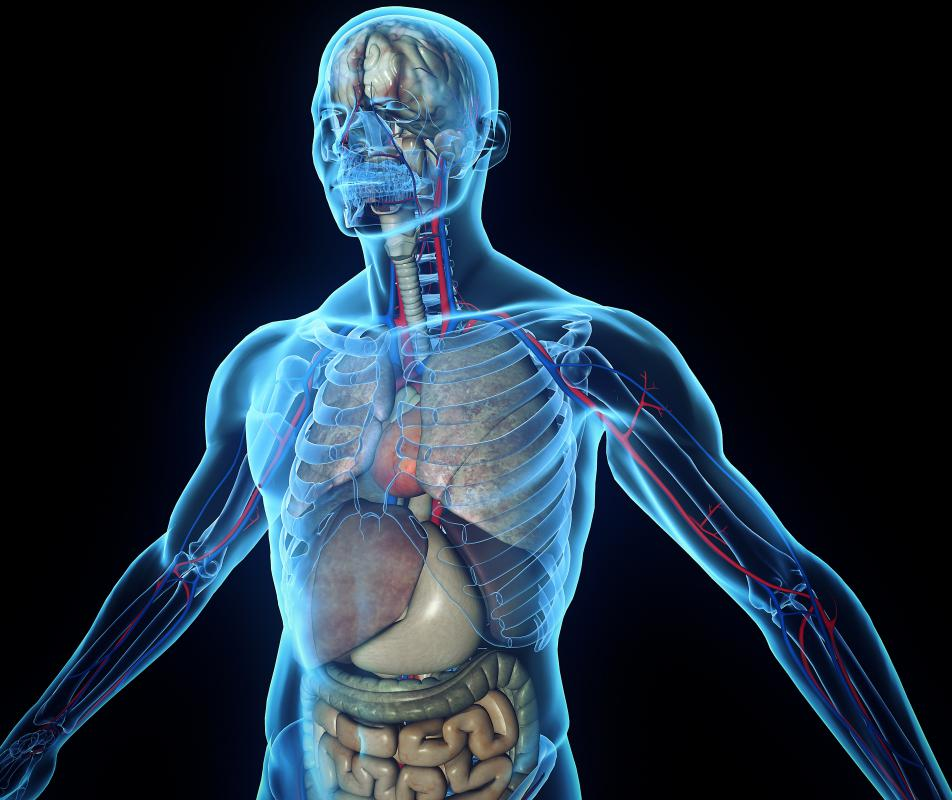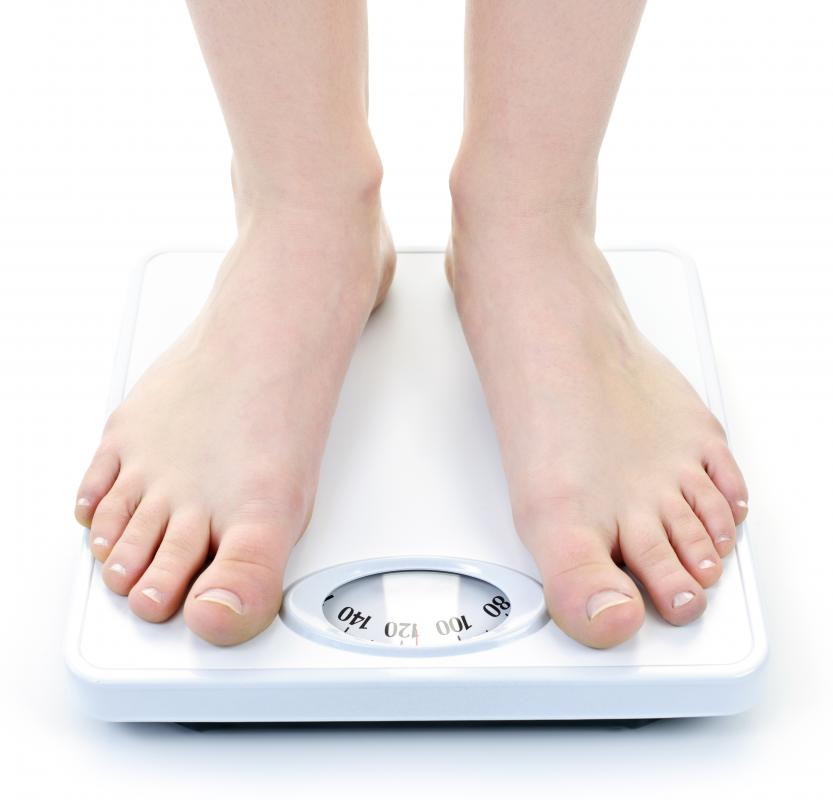# What is Lean Body Weight?

Summer Banks

Lean body weight refers to the total weight of organs, bones, and muscles. All tissues in the body, other than fat, are considered part of lean body mass weight. As body mass increases, so does lean body mass weight, as muscles and organs tend to grow larger as body weight increases.The total weight of organs, muscles, and bones in the body is referred to as lean body weight.

Calculating body weight can be difficult without the proper medical equipment used to measure skeletal, muscle, and organ weight. Men and women have different mathematical equations that are commonly used to determine lean body mass weight. For men who are more than 16 years old: body weight, in kg multiplied by 0.32810, is added to height, in cm, multiplied by 0.33929 to result in a base sum. This sum must then be subtracted by 29.5336, to determine lean body weight.

For a man who weighs 200 pounds (90.71 kg) and measures 6 feet 5 inches (195.6 cm), the following mathematical equation could be used to determine lean body weight.

(90.71 kg x 0.32810) + (195.6 cm x 0.33929) – 29.5336 = 66.59 kg or 146.8 pounds

According to the lean body weight results, 54.2 pounds (24.58 kg) of fat and water make up the rest of this man’s body weight.

Women can also calculate lean body mass weight using a mathematical equation tailored to the female body. For a woman who weighs 135 pounds (61.23 kg) and measures 5 feet 6 inches (167.64 cm), the following equation could be used.

(61.23 kg X 0.29569) + (167.64 cm X 0.41813) – 43.2933 = 44.91 kg or 99 pounds

Lean body mass determined using these equations is an estimate based on a given group of people with a specific height-to-weight ratio. Each person will have a different true lean body weight based on muscle density, bone density, and organ weight. Measuring individual mass requires x-ray technology.

Dual Energy X-ray Absorptiometry (DEXA) uses x-ray to determine body mass and body composition. Resulting measurements often include lean tissue mass, fat tissue mass, and fat percentage. Results can be narrowed down to one of 14 body regions, such as trunk and arms, or reported for total body.

DEXA provides a highly reproducible result which allows physicians to more accurately monitor progress of weight loss and muscle building programs. Even if body weight does not change, lean body mass weight may change due to increased muscle size. Muscle weighs more than fat, so lean body weight increases when muscle growth occurs. Thus, when DEXA is used to measure lean body mass weight, a gain may be healthy rather than a sign of increased fat weight.Men and women have different mathematical equations that are commonly used to determine lean body mass weight.

## You might also Likeanon267617

I am in graduate school and we use a DEXA/DXA for measuring lean body mass as well as bone density. It's the same principle.

flowerchild

@donbri5--I used to call the bone density test a DEXA scan as well. It is actually called a bone densitometry and the abbreviation is DXA, not DEXA. One can get confused when talking about it and using the abbreviation, but they are two different tests, not to be confused.

donbri5

Does anyone know if the DEXA scan mentioned is the same as for bone density testing? My mom is scheduled to have one and I always thought it was to check for bone density, not lean body weight. Thanks

extrordinary

This is very interesting to me because I have been exercising a lot lately. I have noticed a slight difference on the scale, but not much. Interestingly, I have gone down a dress size, so I guess I am getting lean muscle weight! YEA!!